output.to from Sideway
Draft for Information Only

# Content

```Equivalent Couples  Parallel Coplanar Couples Transformation ```

# Equivalent Couples

Equivalent couples can also be formed by the combination of simple transformations.

## Parallel Coplanar Couples Transformation

Two parallel coplanar couples are equal when the force couples can be transfromed by simple couple translation, or forces and couple transformation in space.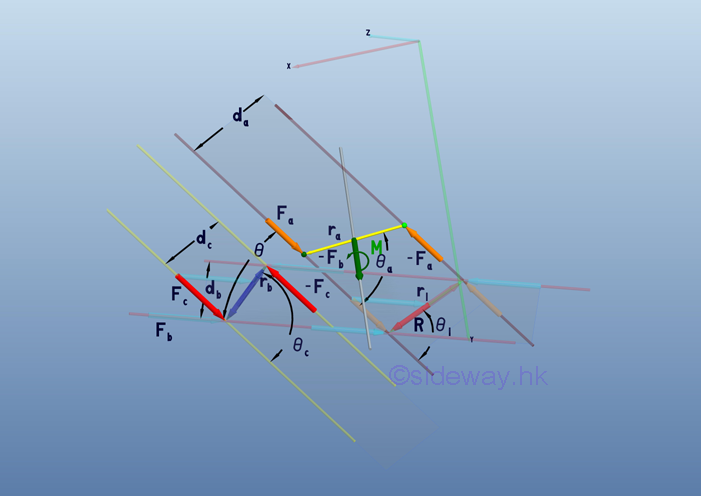By definition, the effective moment of both couples of forces   Fa and  Fc    can be expressed as: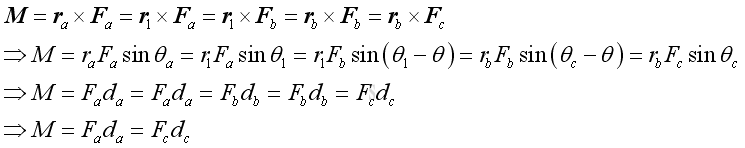For parallel coplanar force couples, two parllel lines can be added at the points of action of the couple of force Fc for forces transmation.  Force Fc can be resolved into force Fb along the parallel line and force along moment arm rc by force decomposition.  By the principle of transmissibility, forces, Fb can be translated along the parallel lines to the intersection of .the line of action of forces Fa to which force Fa can be translated. If two couples of forces  Fa and Fc are equivalent, the vector difference of forces Fa and Fb will aligned with the moment arm vector r1.

By principle of transmissibility, imply moment arms ra and rb can be expressed in terms of r1: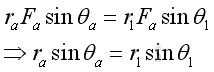and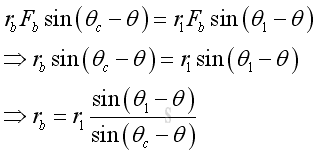Geometrically, prependicular distances da and dc can be expressed as: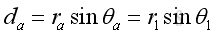and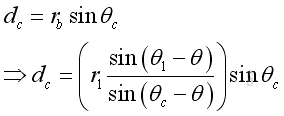Imply the prependicular distance dc can be expressed in terms of da as: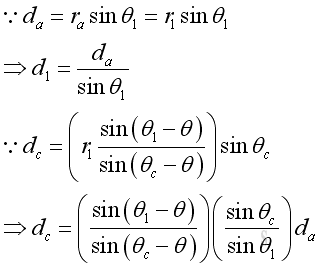By the sine rule, imply force Fb can be expressed in terms of Fa: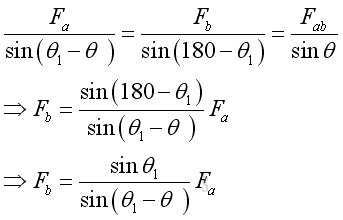By the sine rule, imply force Fc can also be expressed in terms of Fa: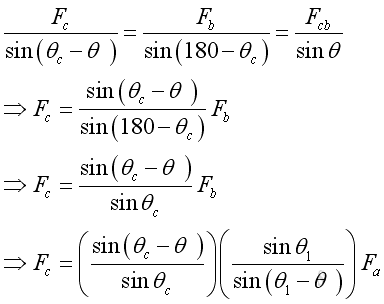Therefore the moment M is: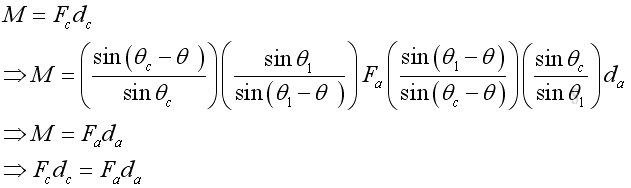Unlike the non-parallel force couples, the angle  c is not a fixed angle and can not be 0o or 180o.

The moment vector M can be expressed as: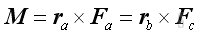Since two force couples are equivalent, they should able to transformed to the same force couple, i.e.  the cross product of moment arm vector r1 or vector rb and the forces Fb . And forces Fa and  Fc  can be expressed in terms of forces Fb . Imply: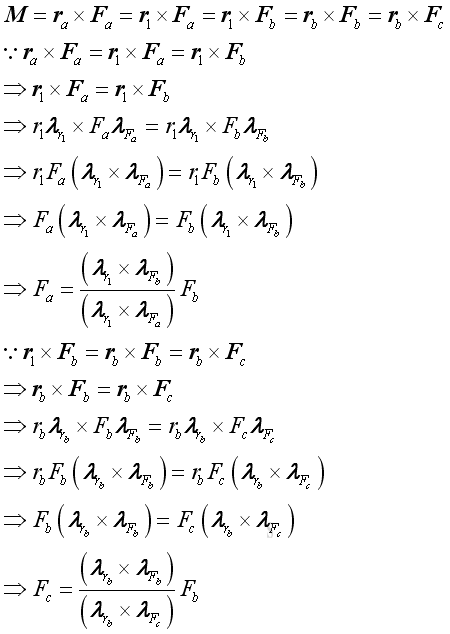Similarly, the moment arm ra and  rb  can also be expressed in terms of moment arm r1 . Imply: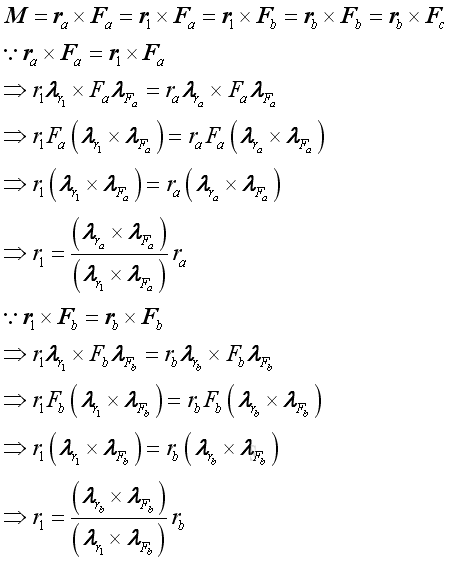Therefore the moment of two couples are equivalent if they can be transformed through the common transformed couple. Imply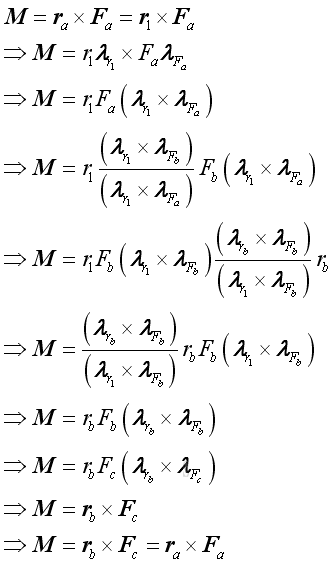Therefore the moment M is: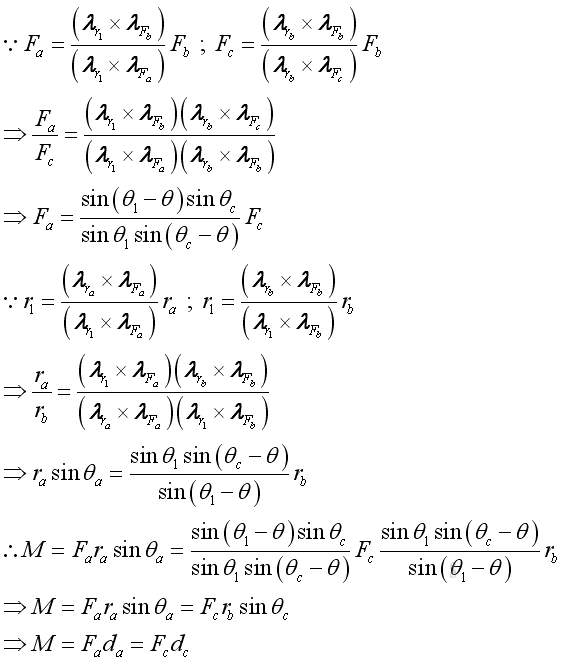ID: 110700001 Last Updated: 7/1/2011 Revision: 0 Ref:References

1. I.C. Jong; B.G. rogers, 1991, Engineering Mechanics: Statics and Dynamics
2. F.P. Beer; E.R. Johnston,Jr.; E.R. Eisenberg, 2004, Vector Mechanics for Engineers: StaticsHome 5

Management

HBR 3

Information

Recreation

Culture

Chinese 1097

English 339

Computer

Hardware 249

Software

Application 213

Latex 52

Manim 205

KB 1

Numeric 19

Programming

Web 289

Unicode 504

HTML 66

CSS 65

SVG 46

ASP.NET 270

OS 429

Python 72

Knowledge

Mathematics

Algebra 84

Geometry 34

Calculus 67

Engineering

Mechanical

Rigid Bodies

Statics 92

Dynamics 37

Control

Natural Sciences

Electric 27